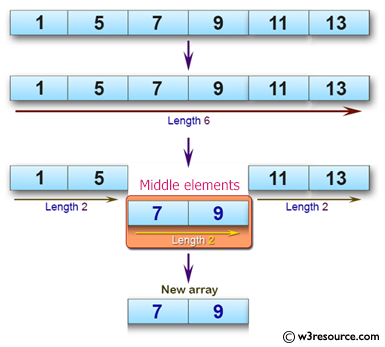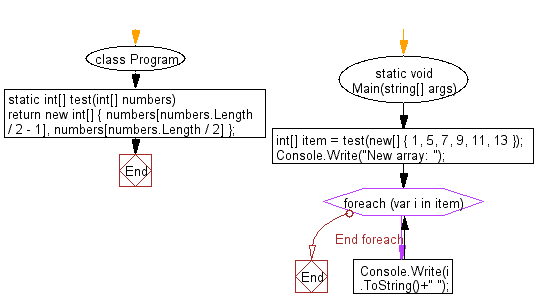﻿ C# - Take two middle elements from an array of even-length# C# Sharp Basic Algorithm Exercises: Create an array taking two middle elements from a given array of integers of length even

## C# Sharp Basic Algorithm: Exercise-103 with Solution

Write a C# Sharp program to create an array taking two middle elements from a given array of integers of length even.

Pictorial Presentation:Sample Solution:-

C# Sharp Code:

``````using System;
namespace exercises
{
class Program
{
static void Main(string[] args)
{
int[] item = test(new[] { 1, 5, 7, 9, 11, 13 });
Console.Write("New array: ");
foreach(var i in item)
{
Console.Write(i.ToString()+" ");
}
}
static int[] test(int[] numbers)
{
return new int[] { numbers[numbers.Length / 2 - 1], numbers[numbers.Length / 2] };
}
}
}
```
```

Sample Output:

`New array: 7 9`

Flowchart:C# Sharp Code Editor:

Improve this sample solution and post your code through Disqus

What is the difficulty level of this exercise?

Test your Programming skills with w3resource's quiz.

﻿